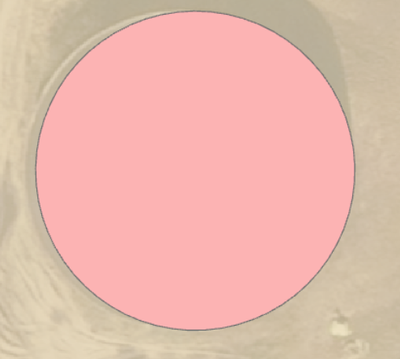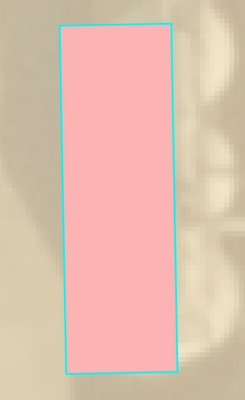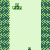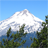# identify the shape of polygon

1003
4
09-01-2021 12:25 AMNew Contributor II

Hi everbody, I have a dataset contains polygon in a geodatabase, some of a shape of a circle like this:and some squard like this:Do you guys know a method, that can identify, what kind of shape the polygons are? I tried count the number of vertex's, which indicate a bit the shape (a high number of vertex indicate a circle - but not always).

Tags (2)
1 Solution

Accepted SolutionsbyMVP Regular Contributor

Hmmm, interesting.

4 vertices and distance(v1, v3) ~ distance(v2, v4): rectangle, else irregular quadrangle.

(Almost) circles: for each vertex, get the distance from the centroid. get min and max distance. if max - min ~ 0: it's a circle, else it's something else.

or: calculate the isoperimetric quotient of the polygon (https://en.wikipedia.org/wiki/Isoperimetric_inequality, https://en.wikipedia.org/wiki/Polsby%E2%80%93Popper_test😞

Q = 4*pi*area/(perimeter^2)

For Q == 1 it's a circle; the lower Q gets, the less circle-ish the shape is.

rectangle-ish shapes with more than 4 vertices: get bearing of each line segment. if bearing[i] ~ bearing[i+1], it's the same side. if you get 4 sides this way, you know its a quadrangle. get the angles between the sides. if they're ~90°, it's a rectangle.

Have a great day!
Johannes
4 RepliesbyMVP Regular Contributor

Hmmm, interesting.

4 vertices and distance(v1, v3) ~ distance(v2, v4): rectangle, else irregular quadrangle.

(Almost) circles: for each vertex, get the distance from the centroid. get min and max distance. if max - min ~ 0: it's a circle, else it's something else.

or: calculate the isoperimetric quotient of the polygon (https://en.wikipedia.org/wiki/Isoperimetric_inequality, https://en.wikipedia.org/wiki/Polsby%E2%80%93Popper_test😞

Q = 4*pi*area/(perimeter^2)

For Q == 1 it's a circle; the lower Q gets, the less circle-ish the shape is.

rectangle-ish shapes with more than 4 vertices: get bearing of each line segment. if bearing[i] ~ bearing[i+1], it's the same side. if you get 4 sides this way, you know its a quadrangle. get the angles between the sides. if they're ~90°, it's a rectangle.

Have a great day!
JohannesbyMVP Notable Contributor

The isoperimetric distance was the first thing I thought of when I saw this post, glad to see it's already been suggested. It'll definitely get you your circles.

For rectangles, you could also orthogonalize the shapes and compare the output against the input. Shapes which changed very little were likely rectangular to begin with.

I have a couple of questions:

1. Are there other shapes besides circles and rectangles you'd like identified? Or were you looking for some way to easily classify your shapes, i.e., building vs. silo? From your screenshots, it looks like you might be dealing with building / silo footprints of some kind.
2. Are there particular tools or processing environments you prefer or avoid? There could be a number of ways to approach this. Model builder, python, Data Interoperability, etc.
- Josh Carlson
Kendall County GISNew Contributor II

Hi Johannes

Thanks, its seams like its working for me, when I use: Q = 4*pi*area/(perimeter^2) like this in field calculater:

(4 * 3.14* !shape.area!) / !shape.length! ** 2

I most interested in, whatever the object is a circle or something else, and if I used Q > 0,98 I get all the circles.byEsri Regular Contributor

Try the following:

Run Minimum Bounding Geometry on your polygons with the CIRCLE geometry type. You will get the smallest circle around every polygon. For input circle like polygons, their Shape_Area would be much closer to the bounding circle than rectangle polygons.

Use Join Field to transfer Shape_Area from the circles to input polygons. The field name will be Shape_Area_1.

Add a field to the input and calculate the ratio between Shape_Area and Shape_Area_1 to the new field. The circle-like polygons should have the ratio values very close to 1. The remaining ones would be rectangle or other non-circle shapes.

Sorry I don't have time to write the details. Hope you get the idea.